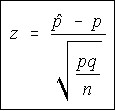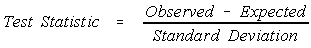Testing a Single Proportion

You are testing p, you are not testing p hat. If you knew the value of p, then there would be nothing to test.

All hypothesis testing is done under the assumption that the null hypothesis is true!

I can't emphasize this enough. The value for all population parameters in the test statistics come from the null hypothesis. This is true not only for proportions, but all of the testing we're going to be doing.The population proportion has an approximately normal distribution if np and nq are both at least 5. Remember that we are approximating the binomial using the normal, and that the p we're talking about is the probability of success on a single trial. The test statistic is shown in the box to the right.

The critical value is found from the normal table, or from the bottom row of the t-table.

The steps involved in the hypothesis testing remain the same. The only thing that changes is the formula for calculating the test statistic and perhaps the distribution which is used.

General Pattern

Notice the general pattern of these test statistics is (observed - expected) / standard deviation.